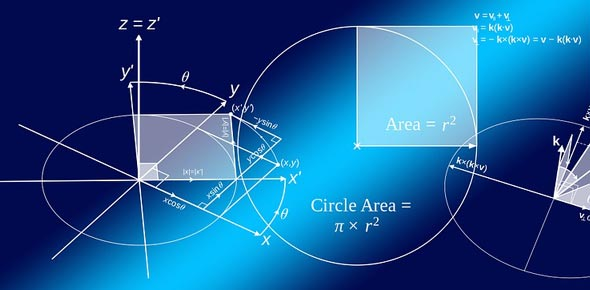7 Questions | Attempts: 145
ShareSettingsYou can check here if you are good at math.

• 1.
Change into a common fraction. 0.125
• A.

1/5

• B.

1/100

• C.

1/8

• D.

1/125

• 2.
Give the name of that equation 5x3=15
• 3.
How many faces does a pentagonal prism have?
• 4.
How many axis of symetry does an equilateral tringle have?
• A.

2

• B.

3

• C.

0

• D.

1

• 5.
How many degrees does the right angle have?
• A.

45

• B.

40

• C.

90

• D.

180

• 6.
Fill in the missing word. minuend- ...........= difference
• A.

Product

• B.

Subtrahend

• C.

• D.

Factor

• 7.
What is the square root of 36?

## Related TopicsBack to top
×

Wait!
Here's an interesting quiz for you.